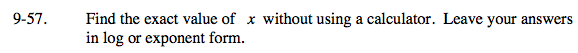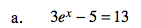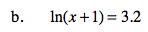### Home > AC > Chapter 16 > Lesson 16.9.1.4 > Problem9-57

9-57.
1. Find the exact value of x without using a calculator. Leave your answers in log or exponent form. Homework Help ✎

1. 3ex − 5 = 13

2. ln(x + 1) = 3.21. Add 5 to both sides.
2. Divide by 3 on both sides.
3. Take the natural log of both sides.
4. Solve for x.e3.2 = x + 1